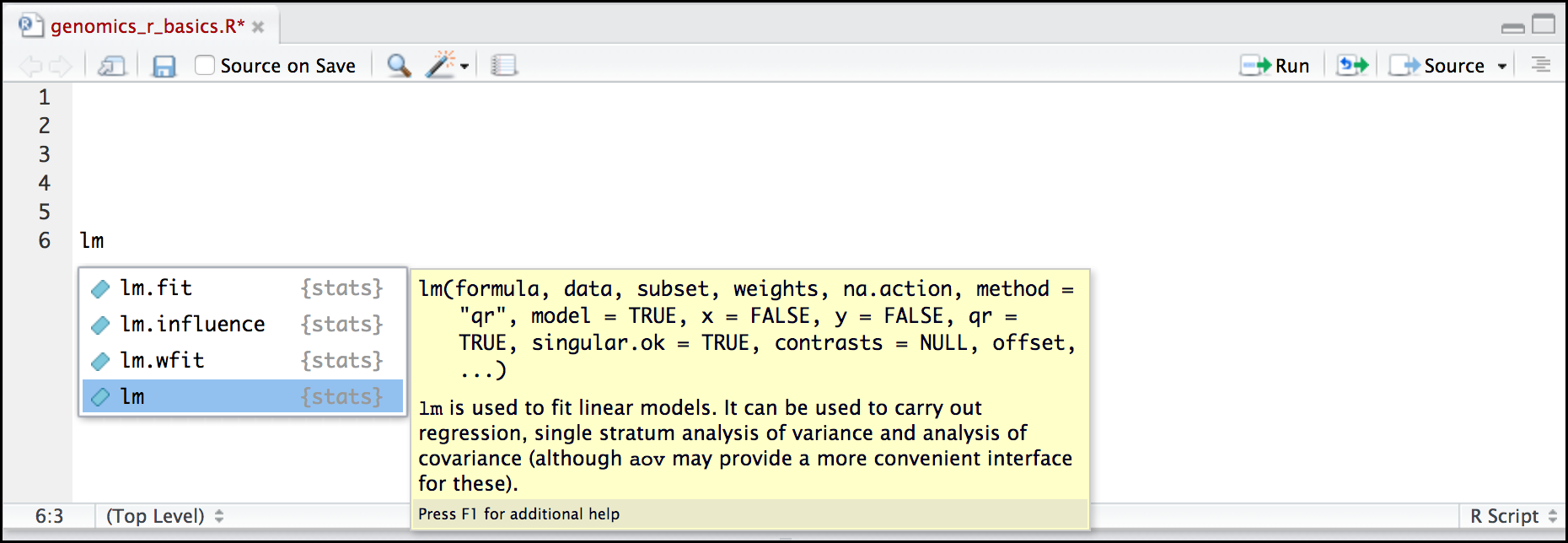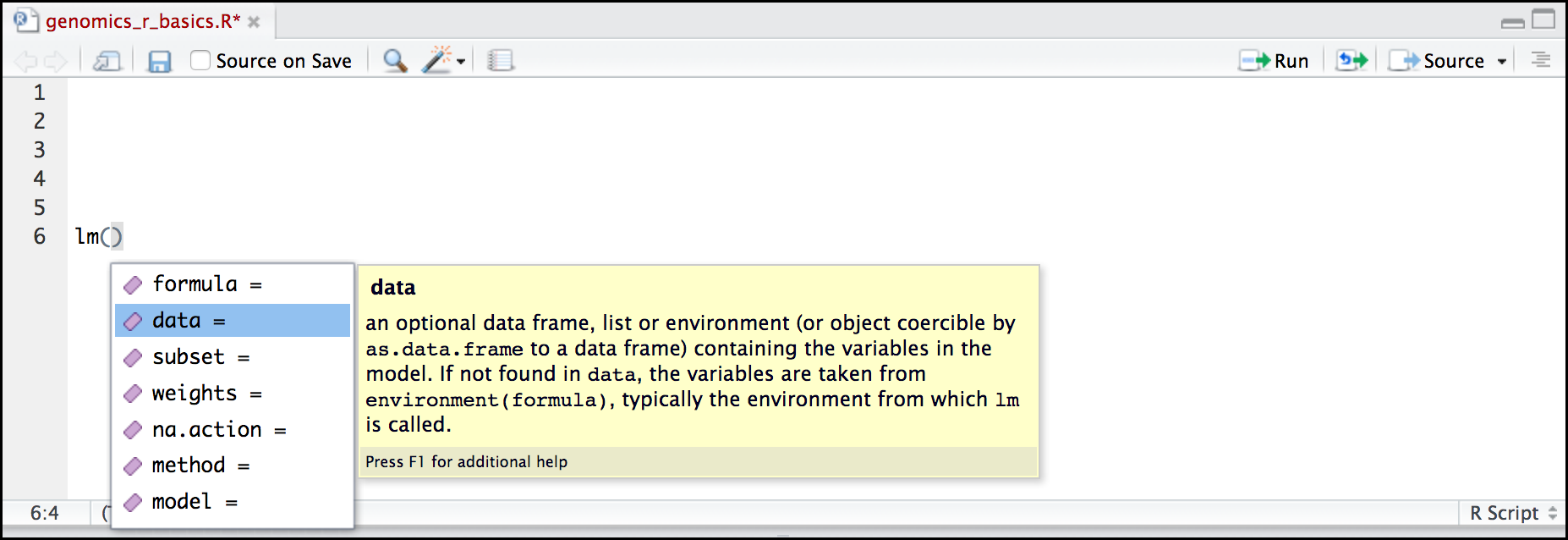# Understanding basic R functions¶

learning-objectives

• “Understand what an R function is”
• “Understand how to modify a function by altering its parameters”
• “Locate help for an R function using ?, ??, and args()

## Using functions in R, without needing to master them¶

A function in R (or any computing language) is a short program that takes some input and returns some output. Functions may seem like an advanced topic (and they are), but you have already used at least one function in R. getwd() is a function! The next sections will help you understand what is happening in any R script.

Question

Exercise: What do these functions do?

• dir()
• sessionInfo()
• date()
• Sys.time()

• dir(): Lists files in the working directory
• sessionInfo(): Gives the version of R and additional info including on attached packages
• date(): Gives the current date
• Sys.time(): Gives the current time

Notice: Commands are case sensitive!

You have hopefully noticed a pattern - an R function has three key properties:

• Functions have a name (e.g. dir, getwd); note that functions are case sensitive!
• Following the name, functions have a pair of ()
• Inside the parentheses, a function may take 0 or more arguments

An argument may be a specific input for your function and/or may modify the function’s behavior. For example the function round() will round a number with a decimal:

```# This will round a number to the nearest integer
round(3.14)
```

## Getting help with function arguments¶

What if you wanted to round to one significant digit? round() can do this, but you may first need to read the help to find out how. To see the help (in R generally called a “vignette”) enter a ? in front of the function name:

```?round()
```

The “Help” tab will show you information (often, too much information). You will slowly learn how to read and make sense of help files. Checking the “Usage” or “Examples” headings is often a good place to look first. If you look under “Arguments,” we also see what arguments we can pass to this function to modify its behavior. You can also see a function’s argument using the args() function:

```args(round)
```

round() takes two arguments, x, which is the number to be rounded, and a digits argument. The = sign indicates that a default (in this case 0) is already set. Since x is not set, round() requires we provide it, in contrast to digits where R will use the default value 0 unless you explicitly provide a different value. We can explicitly set the digits parameter when we call the function:

```round(3.14159, digits = 2)
```

Or, R accepts what we call “positional arguments”, if you pass a function arguments separated by commas, R assumes that they are in the order you saw when we used args(). In the case below that means that x is 3.14159 and digits is 2.

```round(3.14159, 2)
```

Finally, what if you are using ? to get help for a function in a package not installed on your system, such as when you are running a script which has dependencies.

```?geom_point()
```

will return an error:

```Error in .helpForCall(topicExpr, parent.frame()) :
no methods for ‘geom_point’ and no documentation for it as a function
```

Use two question marks (i.e. ??geom_point()) and R will return results from a search of the documentation for packages you have installed on your computer in the “Help” tab. Finally, if you think there should be a function, for example a statistical test, but you aren’t sure what it is called in R, or what functions may be available, use the help.search() function.

Question

Searching for R functions

Use help.search() to find R functions for the following statistical functions. Remember to put your search query in quotes inside the function’s parentheses.

• Chi-Squared test
• Student-t test
• mixed linear model

While your search results may return several tests, we list a few you might find:
• Chi-Squared test: stats::Chisquare
• Student-t test: stats::TDist
• mixed linear model: stats::lm.glm

We will discuss more on where to look for the libraries and packages that contain functions you want to use. For now, be aware that two important ones are CRAN - the main repository for R, and Bioconductor - a popular repository for bioinformatics-related R packages.

## RStudio contextual help¶

Here is one last bonus we will mention about RStudio. It’s difficult to remember all of the arguments and definitions associated with a given function. When you start typing the name of a function and hit the Tab key, RStudio will display functions and associated help:Once you type a function, hitting the Tab inside the parentheses will show you the function’s arguments and provide additional help for each of these arguments.Fix or improve this documentation